Courses

# Analytical Reasoning Quiz III, Non Verbal Reasoning

## 25 Questions MCQ Test Non Verbal Reasoning | Analytical Reasoning Quiz III, Non Verbal Reasoning

Description
This mock test of Analytical Reasoning Quiz III, Non Verbal Reasoning for LR helps you for every LR entrance exam. This contains 25 Multiple Choice Questions for LR Analytical Reasoning Quiz III, Non Verbal Reasoning (mcq) to study with solutions a complete question bank. The solved questions answers in this Analytical Reasoning Quiz III, Non Verbal Reasoning quiz give you a good mix of easy questions and tough questions. LR students definitely take this Analytical Reasoning Quiz III, Non Verbal Reasoning exercise for a better result in the exam. You can find other Analytical Reasoning Quiz III, Non Verbal Reasoning extra questions, long questions & short questions for LR on EduRev as well by searching above.
QUESTION: 1

### Count the number of squares in the given figure.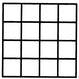Solution:

The figure may be labelled as shown.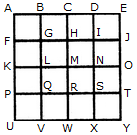The simplest squares are ABGF, BCHG, CDIH, DEJI, FGLK, GHML, HINM, IJON, KLQP, LMRQ, MNSR, NOTS, PQVU, QRWV, RSXW and STYX i.e. 16 in number.

The squares composed of four components each are ACMK, BDNL, CEOM, FHRP, GISQ, HJTR, KMWU, LNXV and MOYW i.e. 9 in number.

The squares composed of nine components each are ADSP, BETQ, FIXU and GJYV i.e. 4 in number.

There is one square AEYU composed of sixteen components.

There are 16 + 9 + 4 + 1 = 30 squares in the given figure.

QUESTION: 2

### Find the number of quadrilaterals in the given figure.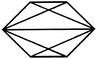Solution:

The figure may be labelled as shown.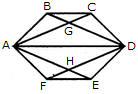The quadrilaterals in the figure are ABCD, ABDE, ABDF, ABDH, CDHA, CDEA, CDFA, DEAG, DEFA, FAGD and AGDH.

The number of quadrilaterals in the figure is 11.

QUESTION: 3

### Count the number of squares in the given figure.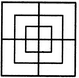Solution:

The figure may be labelled as shown.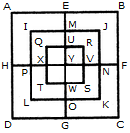The simplest squares are QUYX, URVY, YVSW and XYWT i.e. 4 in number.

The squares composed of two components each are IMYP, MJNY, YNKO and PYOL i.e. 4 in number.

The squares composed of three components each are AEYH, EBFY, YFCG and HYGD i.e. 4 in number.

There is only one square i.e. QRST composed of four components.

There is only one square i.e. IJKL composed of eight components.

There is only one square i.e. ABCD composed of twelve components.

Total number of squares in the given figure = 4 + 4 + 4+1 + 1 + 1 = 15.

QUESTION: 4

What is the minimum number of colours required to fill the spaces in the given diagram without any two adjacent spaces having the same colour?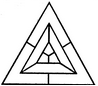Solution:

The figure may be labelled as shown.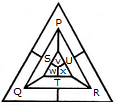The spaces P, Q and R have to be shaded by three different colours definitely (since each of these three spaces lies adjacent to the other two).

Now, in order that no two adjacent spaces be shaded by the same colour, the spaces T, U and S must be shaded with the colours of the spaces P, Q and R respectively.

Also the spaces X, V and W must be shaded with the colours of the spaces S, T and U respectively i.e. with the colours of the spaces R, P and Q respectively. Thus, minimum three colours are required.

QUESTION: 5

Count the number of triangles and squares in the given figure.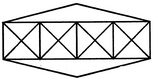Solution:

The figure may be labelled as shown.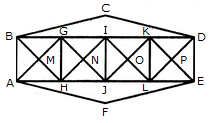Triangles:

The simplest triangles are BGM, GHM, HAM, ABM, GIN, UN, JHN, HGN, IKO, KLO, LJO, JIO, KDP, DEP, ELP, LKP, BCD and AFE i.e. 18 in number.

The triangles composed of two components each are ABG, BGH, GHA, HAB, HGI, GIJ, IJH, JHG, JIK, IKL, KLJ, LJI, LKD, KDE, DEL and ELK i.e. 16 in number.

The triangles composed of four components each are BHI, GJK, ILD, AGJ, HIL and JKE i.e. 6 in number.

Total number of triangles in the figure = 18 +16 + 6 = 40.

Squares :

The squares composed of two components each are MGNH, NIOJ and OKPL i.e. 3 in number.

The squares composed of four components each are BGHA, GIJH, IKLJ and KDEL i.e. 4 in number.

Total number of squares in the figure = 3 + 4 = 7.

QUESTION: 6

Count the number of triangles and squares in the given figure.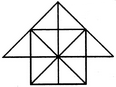Solution:

The figure may be labelled as shown.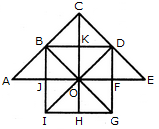Triangles:

The simplest triangles are JBO, BKO, KDO, DFO, FGO, GHO, HIO, IJO, ABJ, BCK, CKD and DEF i.e.12 in number.

The triangles composed of two components each are IBO, BDO, DGO, GIO, ABO, CDO, CBO, CBD and DEO i.e. 9 in number.

The triangles composed of four components each are IBD, BDG, DGI, GIB, ACO and COE i.e. 6 in number.

There is only one. triangle i.e. ACE composed of eight components.

Thus, there are 12 + 9 + 6 + 1 = 28 triangles in the given figure.

Squares:

The squares composed of two components each are BKOJ, KDFO, OFGH and JOHI i.e. 4 in number.

There is only one square i.e. CDOB composed of four components.

There is only one square i.e. BDGI composed of eight components.

Thus, there are 4 + 1 + 1 = 6 squares in the given figure.

QUESTION: 7

What is the minimum number of different colours required to paint he given figure such that no two adjacent regions have the same colour?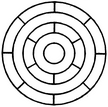Solution:

The figure may be labelled as shown.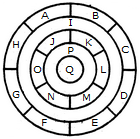The regions A, C, E and G can have the same colour say colour 1.

The regions B, D, F and H can have the same colour (but different from colour 1) say colour 2.

The region 1 lies adjacent to each one of the regions A, B, C, D, E, F, G and H and therefore it should have a different colour say colour 3.

The regions J, L and N can have the same colour (different from colour 3) say colour 1.

The regions K, M and O can have the same colour (different fromthe colours 1 and 3). Thus, these regions will have colour 2.

The region P cannot have any of the colours 1 and 2 as it lies adjacent to each one of the regions J, K, L, M, N and O and so it will have colour 3.

The region Q can have any of the colours 1 or 2.

Minimum number of colours required is 3.

QUESTION: 8

Count the number of parallelogram in the given figure.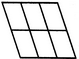Solution:

The figure may be labelled as shown.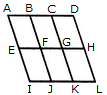The simplest ||gms are ABFE, BCGF, CDHG, EFJI, FGKJ and GHLK. These are 6 in number.

The parallelograms composed of two components each are ACGE, BDHF, EGKI, FHLJ, ABJI, BCKJ and CDLK. Thus, there are 7 such parallelograms.

The parallelograms composed of three components each are ADHE and EHLI i.e. 2 in number.

The parallelograms composed of four components each are ACKI and BDLJ i.e. 2 in number

There is only one parallelogram composed of six components, namely ADLI.

Thus, there are 6 + 7 + 2 + 2 + 1 = 18 parallelograms in the figure.

QUESTION: 9

Count the number of triangles and squares in the given figure.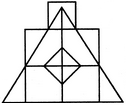Solution:

The figure may be labelled as shown.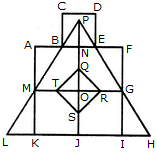Triangles :

The simplest triangles are BPN, PNE, ABM, EFG, MLK, GHI, QRO, RSO, STO and QTO i.e. 10 in number.

The triangles composed of two components each are BPE, TQR, QRS, RST and STQ i.e. 5 in number.

The triangles composed of three components each are MPO and GPO i.e. 2 in number.

The triangles composed of six components each are LPJ, HPJ and MPG i.e. 3 in number.

There is only one triangle LPH composed of twelve components.

Total number of triangles in the figure = 10 + 5 - 2 + 3 + 1 = 21.

Squares :

The squares composed of two components each are KJOM and JIGQ i.e. 2 in number.

The squares composed of three components each are ANOM, NFGO and CDEB i.e.3 in number.

There is only one square i.e. QRST composed of four components.

There is only one square i.e. AFIK composed of ten components.

Total number of squares in the figure = 2 + 3 + 1 + 1 = 7.

QUESTION: 10

Count the number of squares in the given figure.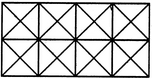Solution:

The figure may be labelled as shown.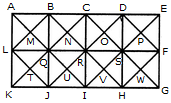The squares composed of two components each are BNQM, CORN, DPSO, MQTL, NRUQ, OSVR, PFWS, QUJT, RVIU and SWHV i.e. 10 in number.

The squares composed of four components each are ABQL, BCRQ, CDSR, DEFS, LQJK, QRIJ, RSHI and SFGH i.e. 8 in number.

The squares composed of eight components each are BRJL, CSIQ and DFHR i.e. 3 in number.

The squares composed of sixteen components each are ACIK, BDHJ and CEGI i.e. 3 in number.

Thus, there are 10 + 8 + 3 + 3 = 24 squares in the figure.

QUESTION: 11

How many triangles and parallelograms are there in the following figure?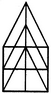Solution:

The figure may be labelled as shown.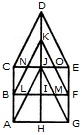Triangles:

The simplest triangles are KJN, KJO, CNB, OEF, JIL, JIM, BLA and MFG i.e. 8 in number.

The triangles composed of two components each are CDJ, EDJ, NKO, JLM, JAH and JGH i.e. 6 in number.

The triangles composed of three components each are BKI, FKI, CJA and EJG i.e. 4 in number.

The triangles composed of four components each are CDE and AJG i.e. 2 in number.

The only triangle composed of six components is BKF.

Thus, there are 8 + 6 + 4 + 2 + 1 = 21 triangles in the given figure.

Parallelograms :

The simplest parallelograms are NJLB and JOFM i.e. 2 in number.

The parallelograms composed of two components each are CDKB, DEFK, BIHA and IFGH i.e.4 in number.

The parallelograms composed of three components each are BKJA, KFGJ, CJIB and JEFI i.e.4 in number.

There is only one parallelogram i.e. BFGA composed of four components.

The parallelograms composed of five components each are CDJA, DEGJ, CJHA and JEGH i.e.4 in number.

The only parallelogram composed of six components is CEFB.

The only parallelogram composed of ten components is CEGA.

Thus, there are 2 + 4 + 4 + 1 + 4+ 1 + 1 = 17 parallelograms in the given figure.

(Here note that the squares and rectangles are also counted amongst the parallelograms).

QUESTION: 12

Count the number of squares in the given figure.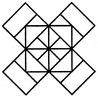Solution:

The figure may be labelled as shown.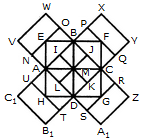The squares composed of two components each are BJMI, CKMJ, DLMK and AIML i.e. 4 in number.

The squares composed of three components each are EBMA, BFCM, MCGD and AMDH i.e. 4 in number.

The squares composed of four components each are VWBA, XYCB, ZA1DC and B1C1AD i.e. 4 in number.

The squares composed of seven components each are NOJL, PQKI, RSLJ and TUIK i.e. 4 in number.

There is only one square i.e. ABCD composed of eight components.

There is only one square i.e. EFGH composed of twelve components.

Total number of squares in the figure = 4 + 4 + 4 + 4 + 1 + 1 = 18.

QUESTION: 13

Count the number of parallelogram in the given figure.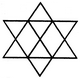Solution:

The figure may be labelled as shown.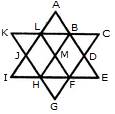The simplest parallelograms are LMHJ and BDFM i.e. 2 in number. The parallelograms composed of two components each are ABML and MFGH i.e. 2 in number.

The parallelograms composed of three components each are LBHI, LBEF, BDGH, DFLA, BCFH, KLFH, A6HJ and LFGJ i.e. 8 in number.

The parallelograms composed of six components each are LCFI, KBEH and ADGJ i.e. 3 in number.

Total number of parallelograms in the figure = 2 + 2 + 8 + 3 = 15.

QUESTION: 14

Count the number of convex pentagons in the adjoining figure.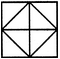Solution:

A convex pentagon has no angles pointing inwards. More precisely, no internal angles can be more than 180�.

The figure may be labelled as shown.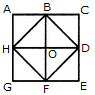The pentagons in the figure, are ABDFH, CDFHB, EFHBD, GHBDF, ACDFG, CEFHA, EGHBC, GABDE, BDEGH, DFGAB, FHACD and HBCEF. Clearly, these are 12 in number.

QUESTION: 15

Count the number of rectangles in the given figure.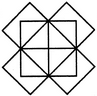Solution:

The figure may be labelled as shown.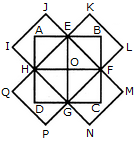The rectangles composed of two components each are HIJE, EKJ,F, FMNG, GPQH, AEOH, EBFO, OFCG and HOGD i.e. 8 in number.

The rectangles composed of four components each are ABFH, BCGE, CDHF, DAEG and EFGH i.e. 5 in number.

The rectangles composed of six components each are IJFG, KLGH, MNHE and PQEF i.e. 4 in number.

The rectangles composed of eight components each are IJMN, KLPQ and ABCD i.e. 3 in number.

Thus, there are 8 + 5 + 4 + 3 = 20 rectangles in the given figure.

(Here note that the squares are also counted amongst rectangles)

QUESTION: 16

Count the number of triangles and squares in the given figure.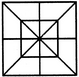Solution:

The figure may be labelled as shown.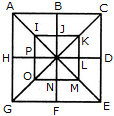Triangles :

The simplest triangles are IJQ, JKQ, KLQ, LMQ, MNQ, NOQ, OPQ and PIQ i.e. 8 in number. The triangles composed of two components each are ABQ, BCQ, CDQ, DEQ, EFQ, FGQ, GHQ, HAQ, IKQ, KMQ, MOQ and OIQ i.e. 12 in number.

The triangles composed of four components each are ACQ, CEQ, EGQ, GAQ, IKM, KMO, MOI and OIK i.e. 8 in number.

The triangles composed of eight components each are ACE, CEG, EGA and GAC i.e. 4 in number.

Total number of triangles in the figure = 8 + 12 + 8 + 4 = 32.

Squares :

The squares composed of two components each are IJQP, JKLQ, QLMN and PQNO i.e. 4 in number.

The squares composed of four components each are ABQH, BCDQ, QDEF and HQFG i.e. 4 in number.

There is only one square i.e. IKMO composed of eight components.

There is only one square i.e. ACEG composed of sixteen components.

Thus, there are 4 + 4 + 1 + 1= 10 squares in the given figure.

QUESTION: 17

Count the number of parallelogram in the given figure.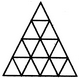Solution:

The figure may be labelled as shown.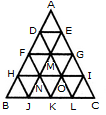The parallelograms composed of two components each are ADME, DFNM, EMOG, FHJN, MNKO, GOLI, HBJN, NJKO, OKLI, FHNM, MNOG, DFME, HJKN, NKLO, OLCI, FNOM, MOIG and DMGE. i.e. 18 in number.

The parallelograms composed of four components each are HOKB, NILJ, FGOH, HOLJ, NICK, FGIN, FMJB, DENH, MGKJ, MGCL, DEIO, FMLK, AENF, AGOD, DMJH, DOKF, EILM and EGKN i.e. 18 in number.

The parallelograms composed of six components each are AEJH, DAIL, DECL, DEJB, HILB and HICJ i.e. 6 in number.

The parallelograms composed of eight components each are FGKB, FGCK and AGKF i.e. 3 in number.

Total number of parallelograms in the figure = 18 + 18 + 6 + 3 = 45.

QUESTION: 18

Count the number of parallelogram in the given figure.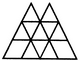Solution:

The figure may be labelled as shown.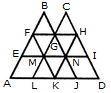The simplest parallelograms are EMLA and NIDJ i.e. 2 in number.

The parallelograms composed of two components each are BFMG, CGNH, GMKN, FGME, GHNM, MNKL, FGNM, GHIN and MNJK i.e. 9 in number.

The parallelograms composed of three components each are FGLA, ENKA, GHDJ and MIDK ie. 4 in number.

The parallelograms composed of four components each are FGJK, GHKL, FBNK, CHKM, EFHN and MFHI i.e. 6 in number.

The parallelograms composed of seven components each are FHKA and FHDK i.e. 2 in number.

Total number of parallelograms in the figure = 2 + 9 + 4 + 6 + 2 = 23.

QUESTION: 19

Count the number of squares in the given figure.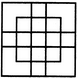Solution:

The figure may be labelled as shown.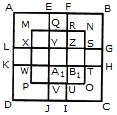The simplest squares are EFRQ, MQYX, QRZY, RNSZ, LXWK, XYA1W, YZB1A1, ZSTB1, SGHT, WA1VP, A1B1UV, B1TOU and VUIJ i.e. 13 in number.

The squares having two components each are AEYL, FBGZ, KA1JD and B1HCI i.e. 4 in number.

The squares having four components each are MRB1W, QNTA1 XZUP and YSOV i.e. 4 in number.

The squares having seven components each are AFB1K, EBHA1 LZID and YGCJ i.e. 4 in number.

There is only one square i.e. MNOP composed of nine components.

There is only one square i.e. ABCD composed of seventeen components.

There are 13+ 4 + 4 + 4+1 + 1 = 27 squares in the figure.

QUESTION: 20

What is the minimum number of straight lines that is needed to construct the figure?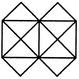Solution:

The figure may be labelled as shown.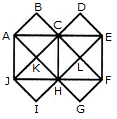The horizontal lines are AE and JF i.e. 2 in number. The vertical lines are AJ, CH and EF i.e. 3 in number.

The slanting lines are AG, BF, JD, IE, AB, DE, JI and FG i.e. 8 in number.

Total number of straight lines needed to construct the figure = 2 + 3 + 8 = 13.

QUESTION: 21

How many rectangles are there in the given figure.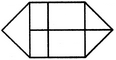Solution:

The figure may be labelled as shown.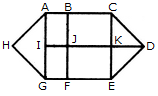The simplest rectangles are ABJI, BCKJ, IJFG and JKEF i.e. 4 in number.

The rectangles composed of two components each are ACKI, BCEF, IKEG and ABFG i.e. 4 in number.

The only rectangle composed of four components is ACEG.

Thus, there are 4 + 4 + 1 = 9 rectangles in the given figure.

QUESTION: 22

Determine the number of rectangles and hexagons in the given figure.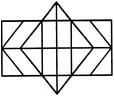Solution:

The figure may be labelled as shown.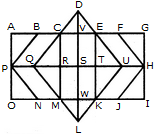Rectangles:

The simplest rectangles are CVSR, VETS, RSWM and STKW i.e. 4 in number.

The rectangles composed of two components each are CETR, VEKW, RTKM and CVWM i.e. 4 in number.

The rectangles composed of three components each are ACRP, PRMO, EGHT and THIK i.e. 4 in number.

The rectangles composed of four components each are CEKM, AVSP,PSWO, VGHS and SHIW i.e. 5 in number.

The rectangles composed of five components each are AETP, PTKO, CGHR and RHIM i.e. 4 in number.

The rectangles composed of six components each are ACMO and EGIK i.e. 2 in number.

The rectangles composed of eight components each are AGHP, PHIO, AVWO and VGIW i.e. 4 in number.

The rectangles composed of ten components each are AEKO and CGIM i.e. 2 in number.

AGIO is the only rectangle having sixteen components.

Total number of rectangles in the given figure

= 4 + 4 + 4 + 5 + 4 + 2 + 4 + 2 + 1 = 30.

Hexagons:

The hexagons in the given figure are CDEKLM, CEUKMQ, CFHJMQ, BEUKNP and BFHJNP.

So, there are 5 hexagons in the given figure.

QUESTION: 23

Count the number of triangles and squares in the given figure.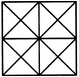Solution:

The figure may be labelled as shown.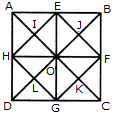Triangles :

The simplest triangles are AEI, EOI, OHI, HAI, EBJ, BFJ, FOJ, OEJ, HOL, OGL, GDL, DHL, OFK, FCK, CGK and GOK i.e. 16 in number.

The triangles composed of two components each are HAE, AEO, EOH, OHA, OEB, EBF, BFO, FOE, DHO, HOG, OGD, GDH, GOF, OFC, FCG and CGO i.e. 16 in number.

The triangles composed of four components each are HEF, EFG, FGH, GHE, ABO, BGO, CDO and DAO i.e. 8 in number.

The triangles composed of eight components each are DAB, ABC, BCD and CDA i.e. 4 in number.

Total number of triangles in the figure = 16 + 16 + 8 + 4 = 44.

Squares :

The squares composed of two components are HIOL, IEJO, JFKO and KGLO i.e. 4 in number.

The squares composed of four components are AEOH, EBFO, OFGC and HOGD i.e.4 in number.

There is only one square EFGH which is composed of eight components.

There is only one square ABCD which is composed of sixteen components.

Total number of squares in the figure = 4 + 4 + 1 + 1 = 10.

QUESTION: 24

Count the number of rectangles in the given figure.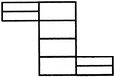Solution:

The figure may be labelled as shown.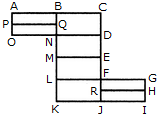The simplest rectangles are ABQP, PQNO, BCDN, NDEM, MEFL, LFJK, FGHR and RHIJ i.e. 8 in number.

The rectangles composed of two components each are ABNO, BCEM, NDFL, MEJK and FGIJ i.e. 5 in number.

The rectangles composed of three components each are ACDO, BCFL, NDJK and LGIK i.e. 4 in number.

There is only one rectangle i.e. BCJK composed of four components.

Total number of rectangles in the figure = 8 + 5 + 4 + 1 = 18.

QUESTION: 25

Count the number of squares in the given figure.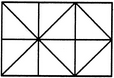Solution:

The figure may be labelled as shown.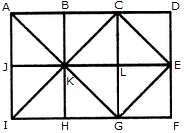The squares composed of two components each are ABKJ, BCLK, CDEL, LEFG, KLGH and JKHI i.e.6 in number.

There is only one square i.e. CEGK composed of four components.

The squares composed of eight components each are ACGI and BDFH i.e. 2 in number.

There are 6 + 1 + 2 = 9 squares in the figure.